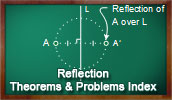# Online Math: Geometry: Reflection, Flip, Theorems and Problems

 Reflection - IndexGeometry Problem 1143. Triangle, Circumcircle, Tangent Line, Reflection of a Point over a Line, Tangent Circles, Collinear Points. Geometry Problem 1059. Triangle, Circumcircle, Orthocenter, Altitude, Perpendicular, Reflection of a Point over a Line, Collinear Points, Simson Line, Congruence. Geometry Problem 974. 'Begonia Theorem', Cevian Triangle, Reflection of a point in a line, Concurrency of Lines. GeoGebra, HTML5 Animation for Tablets. Geometry Problem 929. Triangle, Orthocenter, Circumcircle, Reflection, Concurrency. Geometry Problem 733. Triangle, Orthocenter, Altitude, Reflection in a line, Circumcircle, Concurrency.